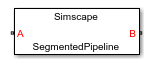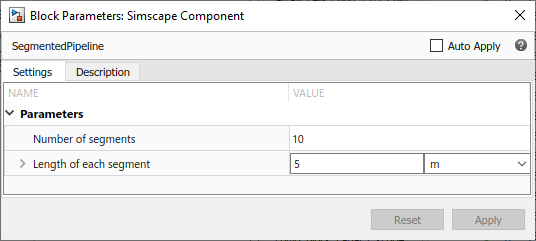## Segmented Pipeline Using Component Array

This example shows how you can model a segmented pipeline using a component array. This segmented pipeline model is a composite component that consists of `N` identical segments connected in series. Individual pipe segments are represented by the Pipe (IL) blocks from the Foundation library. `N` is a parameter that the block user can modify.

```component SegmentedPipeline parameters N = 10; % Number of segments segm_length = { 5, 'm' }; % Length of each segment end % Ports at the two ends of the pipeline nodes A = foundation.isothermal_liquid.isothermal_liquid; % A:left B = foundation.isothermal_liquid.isothermal_liquid; % B:right end % Declare array of N components for i=1:N components (ExternalAccess=none) pipe(i) = foundation.isothermal_liquid.elements.pipe(length = segm_length); end end % Connect all segments in series for i=1:(N-1) connections connect(pipe(i).B, pipe(i+1).A); end end % Connect two ends of pipeline to first and last segment, respectively connections connect(A, pipe(1).A); connect(B, pipe(N).B); end end```

In this example, for the sake of simplicity, the `SegmentedPipeline` component has only two modifiable parameters: `N` (Number of segments) and `segm_length` (Length of each segment). However, you can make other parameters of the underlying Pipe (IL) block accessible from the top-level composite component block dialog, as described in Parameterizing Composite Components. Parameter `N`, which defines the array size and is going to be used as the upper limit for the `for`-loop iterator, is declared as a unitless integer.

Use a `for`-loop to declare an array of `N` member components:

``` for i=1:N components (ExternalAccess=none) pipe(i) = foundation.isothermal_liquid.elements.pipe(length = segm_length); end end ```

In this example, all the pipe segments have the same length. For examples of array component members having different parameter values, see Segmented Pipeline with Different Pipe Lengths Using Component Array and Case Study — Battery Pack with Fault Using Arrays.

Use another `for`-loop to connect all segments in series, by connecting node `B` of each pipe segment (except the last one) to node `A` of the next segment:

``` for i=1:(N-1) connections connect(pipe(i).B, pipe(i+1).A); end end ```

Finally, connect the internal chain of segments to the two ends of pipeline, by connecting node `A` of the composite component to node `A` of the first segment and connecting node `B` of the composite component to node `B` of the last segment:

``` connections connect(A, pipe(1).A); connect(B, pipe(N).B); end end ```

The resulting block has two isothermal liquid ports, A and B, and two modifiable parameters: Number of segments and Length of each segment.